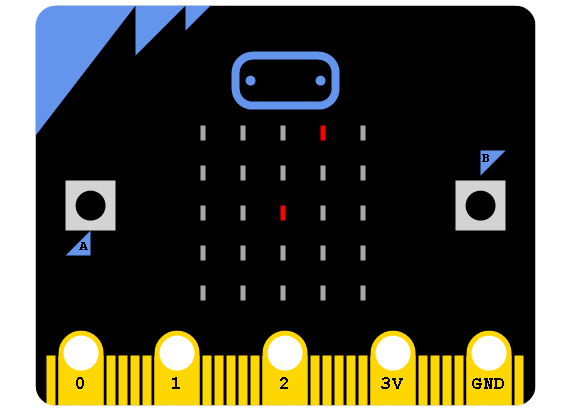# BBC micro:bit Knight Moves

## Introduction

The inspiration for this project is an old chess-style puzzle called Knight's Tour. The idea is to place a knight on a square of a chessboard and then, using only knight moves, visit every square on the board exactly once.

On the micro:bit, each dot on the matrix will represent a square on a 5x5 chessboard. In the image below, the two red dots show one way that a knight might move from the centre spot.## Programming

I did this in two stages. The first was to allow the user to pick a spot and then have all the reachable destinations shown on the matrix.

### Showing The Moves

I wanted to be able to think about the matrix as a series of numbers from 0 to 24 as well as a 5x5 grid. The first two functions, xy2idx and idx2xy perform that conversion.

The MovesFromSpot function does the work. The mvs list stores the 8 possible changes to our grid position. Each one of these changes that stays within the grid is a move.

I used the user interface code from Lights Out - tilt the micro:bit to move the dot, press the A button to show the moves.

```from microbit import *

def xy2idx(x1,y1):
return y1*5+x1

def idx2xy(idx):
return idx%5,idx // 5

def DrawGame(t,x,y):
img = Image('00000:'*5)
img.set_pixel(x, y, (t % 2)*9)
return img

def MovesFromSpot(s):
xx,yy = idx2xy(s)
mvs = [(-1, -2),( 1, -2),(-2, -1),( 2, -1),
(-2,  1),( 2,  1),(-1,  2), ( 1,  2)]
r = range(5)
result = []
for m in mvs:
dx,dy = m
nx = xx + dx
ny = yy + dy
if nx in r and ny in r:
result.append(xy2idx(nx,ny))
return result

def ShowMoves(x1,y1):
display.clear()
display.set_pixel(x1,y1,9)
mm = MovesFromSpot(xy2idx(x1,y1))
for p in mm:
px,py = idx2xy(p)
display.set_pixel(px,py,5)
sleep(5000)
display.clear()

x = 2
y = 2
tick = -1

while True:
tick +=1
if tick==2:
tick = 0
# check for movement
dx = accelerometer.get_x()
dy = accelerometer.get_y()
if dx > 300:
x += 1
sleep(200)
if dx < -300:
x -= 1
sleep(200)
if dy > 300:
y += 1
sleep(200)
if dy < -300:
y -= 1
sleep(200)
# keep on grid
x = max(0, min(x, 4))
y = max(0, min(y, 4))
# update screen
i = DrawGame(tick,x,y)
display.show(i)
if button_a.was_pressed():
ShowMoves(x,y)
sleep(50)
```

### Touring

There are many ways to approach this problem. One approach is called Warnsdorf's rule, after its inventor. Warnsdorf's rule is a heuristic. A heuristic is a practical method for finding a sub-optimal solution to a problem. According to the rule, each new move should be chosen to take the knight to the square with the least number of onward moves.

The tour is implented by adding a function that is called when the B button is pressed. A buzzer was attached to pin0 for some sound effects.

```from microbit import *
import music
import random

def xy2idx(x1,y1):
return y1*5+x1

def idx2xy(idx):
return idx%5,idx // 5

def DrawGame(t,x,y):
img = Image('00000:'*5)
img.set_pixel(x, y, (t % 2)*9)
return img

def MovesFromSpot(s):
xx,yy = idx2xy(s)
mvs = [(-1, -2),( 1, -2),(-2, -1),( 2, -1),
(-2,  1),( 2,  1),(-1,  2), ( 1,  2)]
r = range(5)
result = []
for m in mvs:
dx,dy = m
nx = xx + dx
ny = yy + dy
if nx in r and ny in r:
result.append(xy2idx(nx,ny))
return result

def ShowMoves(x1,y1):
display.clear()
display.set_pixel(x1,y1,9)
mm = MovesFromSpot(xy2idx(x1,y1))
for p in mm:
px,py = idx2xy(p)
display.set_pixel(px,py,5)
sleep(5000)
display.clear()

def TourFrom(x1,y1):
display.clear()
display.set_pixel(x1,y1,9)
current = xy2idx(x1,y1)
touring = True
visited = []
while touring:
visited.append(current)
if len(visited)==25:
music.play(music.BA_DING)
touring = False
else:
# get all moves from here
allmvs = MovesFromSpot(current)
# filter to unvisited spots
vms = [i for i in allmvs if i not in visited]
if len(vms)==0:
# no moves left - fail
music.pitch(440,3000)
touring = False
else:
# find next best move
# get number of moves at each possible destination
lgths = []
for m in vms:
tmp = MovesFromSpot(m)
tmp1 = [i for i in tmp if i not in visited]
lgths.append(len(tmp1))
lwst = min(lgths)
# candidates for a move
tm = [p for i,p in enumerate(vms) if lgths[i]==lwst]
current = random.choice(tm)
x2,y2 = idx2xy(current)
display.set_pixel(x2,y2,5)
music.pitch(440,50)
sleep(50)
print(visited)

x = 2
y = 2
tick = -1

while True:
tick +=1
if tick==2:
tick = 0
# check for movement
dx = accelerometer.get_x()
dy = accelerometer.get_y()
if dx > 300:
x += 1
sleep(200)
if dx < -300:
x -= 1
sleep(200)
if dy > 300:
y += 1
sleep(200)
if dy < -300:
y -= 1
sleep(200)
# keep on grid
x = max(0, min(x, 4))
y = max(0, min(y, 4))
# update screen
i = DrawGame(tick,x,y)
display.show(i)
if button_a.was_pressed():
ShowMoves(x,y)
if button_b.was_pressed():
TourFrom(x,y)
sleep(50)
```

## Review

The program does not find a path for all starting squares. Tours are not possible from the following starting points,

• 0,1
• 0,3
• 1,0
• 1,2
• 1,4
• 2,1
• 2,3
• 3,2
• 3,4
• 4,1
• 4,3

It is also possible for the program to fail to find a tour from some of the other starting squares. When choosing a move, sometimes there is a tie between two or more reachable destinations. This code chooses randomly between them. Other approaches to the tiebreak include choosing to move to the point furthest from the centre.

Rather than watch the dot walking around, you could adapt the program so that it has several goes at solving for the squares we know can be solved.

There is a game to be made out of this. Generate a random starting point from the list of spots you know work. Have the user choose the moves and try to solve the puzzle.